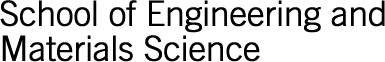Close# School of Engineering and Materials Science Research Student Awards

## PhD Thesis: Model Identification and Robust Nonlinear Model Predictive Control of a Twin Rotor MIMO System

Author: RAHIDEH, Akbar

Year: 2009

Supervisor(s): Hasan Shaheed

This thesis presents an investigation into a number of model predictive control (MPC) paradigms for a nonlinear aerodynamics test rig, a twin rotor multi-input multi-output system (TRMS). To this end, the nonlinear dynamic model of the system is developed using various modelling techniques. A comprehensive study is made to compare these models and to select the best one to be used for control design purpose. On the basis of the selected model, a state-feedback multistep Newton-type MPC is developed and its stability is addressed using a terminal equality constraint approach. Moreover, the state-feedback control approach is combined with a nonlinear state observer to form an output-feedback MPC. Finally, a robust MPC technique is employed to address the uncertainties of the system.

In the modelling stage, analytical models are developed by extracting the physical equations of the system using the Newtonian and Lagrangian approaches. In the case of the black-box modelling, artificial neural networks (ANNs) are utilised to model the TRMS. Finally, the grey-box model is used to enhance the performance of the white-box model developed earlier through the optimisation of parameters using a genetic algorithm (GA) based approach. Stability analysis of the autonomous TRMS is carried out before designing any control paradigms for the system.

In the control design stage, an MPC method is proposed for constrained nonlinear systems, which is the improvement of the multistep Newton-type control strategy. The stability of the proposed state-feedback MPC is guaranteed using terminal equality constraints. Moreover, the formerly proposed MPC algorithm is combined with an unscented Kalman filter (UKF) to formulate an output-feedback MPC. An extended Kalman filter (EKF) based on a state-dependent model is also introduced, whose performance is found to be better compared to that of the UKF. Finally, a robust MPC is introduced and implemented on the TRMS based on a polytopic uncertainty that is cast into linear matrix inequalities (LMI).